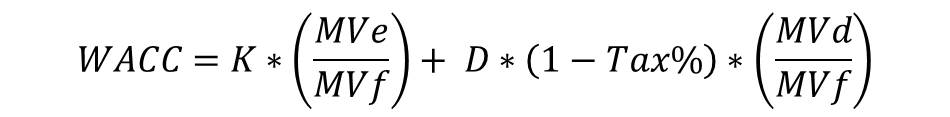data-full-width-responsive="true">

# WACC Calculator

## What is Weighted Average Cost of Capital (WACC)?

Weighted average cost of capital (WACC) is the overall cost of capital of a firm derived by a weighted average of cost of equity, preferred and debt capital. Weights are nothing but the respective weight of each type of capital.

## WACC Formula

The mathematical expression of the formula of WACC is as follows: